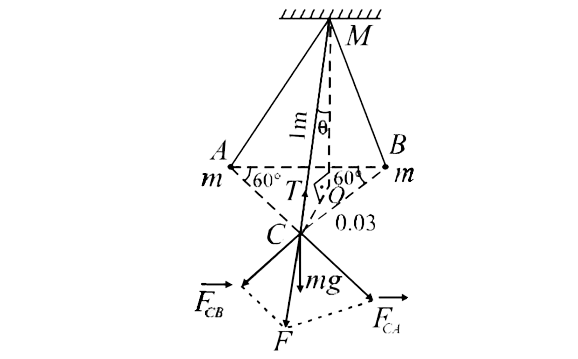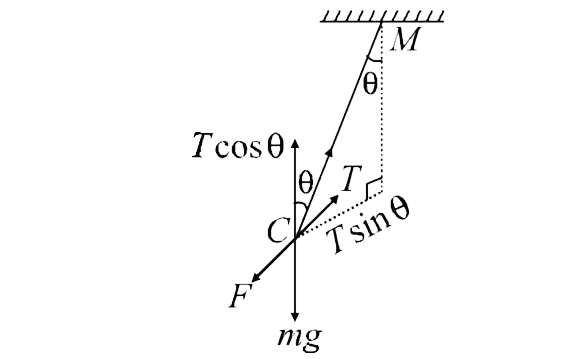Question

Updated on:26/04/2023

# Three particles, each of mass 1gm and carrying a charge q, are suspended from a common point by insulated massless strings, each 100cm long. If the particles are in equilibrium and are located at the corners of an equilateral triangle of side length 3cm, calculate the charge q on each particle. (Take g=10m/s2).

Text Solution

Solution
Each mass will be in equilibrium under the act of three force namely tension of string, weight, resultant electrostatic force of the two other charges out of these three forces F and mg are perpendicular.Let T make and angle θ with the vertical
OC=23(0.03)2(0.015)2=0.0173m
OM=0.9997
NOTE THIS STEP: Resolving T in the direction of mg and F and applying the condition of equilibrium, we get
Tcosθ=mg, Tsinθ=F
tanθ=Fmg ...(i)
F=F2CA+F2CB+2FCAFCBcosα
F=F2CA+F2CA+2F2CA×12
F=3FCA=3×kq2(CA)2 ...(ii)
[where FCB=ForceonCdueB<br>FCA=ForceonCdueA<br>|vecF_(CB)|=|vecF_(CA)|andalpha=60^@]<br>Also,tantheta=(OC)/(OM)=(0.0173)/(0.9997)...(iii)<br>From(i),(ii)and(iii)<br>(0.0173)/(0.9997)=(sqrt3xx9xx10^9xxq^2)/((0.03)^2xx10^-3xx9.8)<br>Onsolvg,wetq=3.16xx10^-9C.